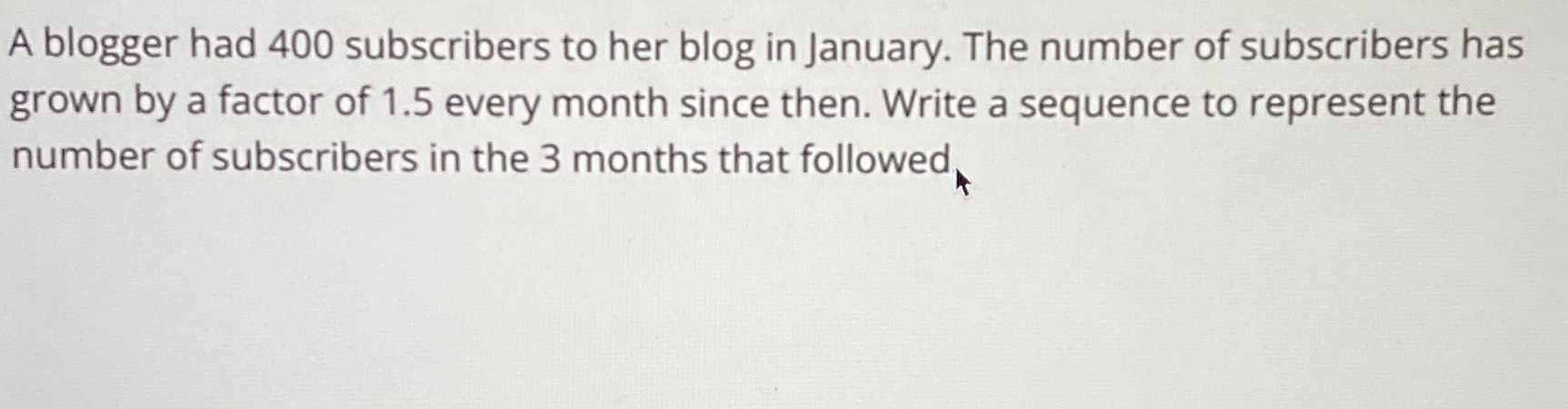### ¿Todavía tienes preguntas de matemáticas?

Pregunte a nuestros tutores expertos
Algebra
PreguntaA blogger had $$400$$ subscribers to her blog in January. The number of subscribers has grown by a factor of $$1.5$$ every month since then. Write a sequence to represent the number of subscribers in the $$3$$ months that followed

$$1350$$